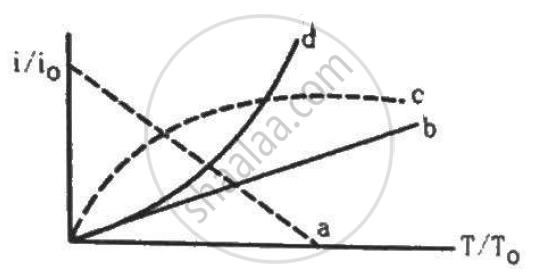Department of Pre-University Education, Karnataka course PUC Karnataka Science Class 12
Share

# Let I0 Be the Thermionic Current from a Metal Surface When the Absolute Temperature of the Surface is T0. - Physics

ConceptElectron Emission

#### Question

Let i0 be the thermionic current from a metal surface when the absolute temperature of the surface is T0. The temperature is slowly increased and the thermionic current is measured as a function of temperature. Which of the following plots may represent the variation in (i/i0) against (T/T0)?• a

• b

• c

• d

#### Solution

d

Since the thermionic current is directly proportional to the square of the temperature of the surface emitting thermions, the graph is parabolic.

Is there an error in this question or solution?

#### Video TutorialsVIEW ALL 

Solution Let I0 Be the Thermionic Current from a Metal Surface When the Absolute Temperature of the Surface is T0. Concept: Electron Emission.
S